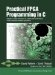# CO_BIT_EXTRACT_U

` int32 co_bit_extract_u(uint32 value,                        uint8 start_bit,                        uint8 num_bits); `

co.h

#### Description

This function provides a concise and efficient method of extracting bits from a (32-bit) unsigned integer.

#### Arguments

The arguments for co_bit_extract_u are as follows:

 uint32 value The 32-bit unsigned integer from which the bits are taken. uint8 start_bit The starting bit (LSB) of the extracted bit sequence. uint8 num_bits The number of bits to extract.

#### Return Value

Returns a 32-bit unsigned integer (uint32) value representing the value of the extracted bits.

#### Notes

The co_bit_insert, co_bit_insert_u, co_bit_extract, and co_bit_extract_u functions operate on 32-bit data. If required, you can truncate the input and results to suit your needs. (Types larger than 32 bits are currently not supported.) For example, if you are operating on 16-bit numbers, you can use the functions as follows:

` co_int16 src = 0xffff; co_int16 dest; dest = co_bit_extract(src, 8, 16); // dest == 0x00ff dest = co_bit_insert(src, 8, 8, dest); // dest == 0xffff `

In this example, src and dest are automatically promoted (and sign-extended, since they are signed types) to 32-bit integers when used as arguments, and the return value gets cast invisibly to a co_int16, which just truncates 32 to 16 bits.

The difference between signed and unsigned bit extractions (co_bit_extract and co_bit_extract_u, respectively) has to do with what's beyond the most-significant bit. If you try to extract 16 bits from signed value 0xffff, starting (halfway) at bit 8, you get 0xffff. If you extract the same bits from unsigned 0xffffU, you get 0x00ffU, since the sign is not extended beyond the existing 16 bits. Otherwise, the extract functions do the same thing.Practical FPGA Programming in C
ISBN: 0131543180
EAN: 2147483647
Year: 2005
Pages: 208

Similar book on Amazon